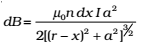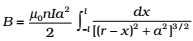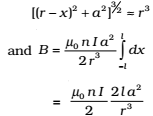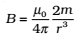To demonstrate the similarity of a current carrying solenoid to a bar magnet, let us calculate axial field of a finite solenoid carrying current.Consider:        a = radius of solenoid

2l = length of solenoid with centre O

n = number of turns per unit length

I = current passing through solenoid

OP = r

Consider a small element of thickness dx of solenoid at distance x from O. and number of turns in element = n dx.

We know magnetic field due to n turns coil at axis of solenoid is given byThe magnitude of the total field is obtained by summing over all the elements — in other words by integrating from x = – l to x = + l. Thus,This integration can be done by trigonometric substitutions. This exercise, however, is not necessary for our purpose. Note that the range of x is from – l to + l. Consider the far axial field of the solenoid, i.e., r >> a and r >> l. Then the denominator is approximated byNote that the magnitude of the magnetic moment of the solenoid is, m = n (2l) I (a2) — (total number of turns × current × cross-sectional area). Thus,It is clear from the above expression that magnetic moment of a bar magnet is equal to the magnetic moment of an equivalent solenoid that produces the same magnetic field.